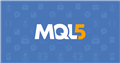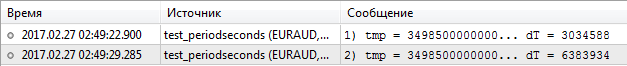# Questions from Beginners MQL5 MT5 MetaTrader 5 - page 1379

User_mt5 #:

The Bible says: The _Period variable stores the timeframe value of the current chart.

The actual values of _Period for the TF:

М1 = 1

М5 = 5

М15 = 15

М30 = 30

H1 = 16385

H4 = 16388

H6 = 16390

Explain please, the numbers 60, 120 etc. are... no longer in vogue?

If you know, at least give me a hint as to what the trick is here?

And now I'm afraid to ask ... where else in our mysterious MQL are such tricks? :(

here are the periodshttps://www.mql5.com/ru/docs/constants/chartconstants/enum_timeframes

\\\\\\\\\\\\\\\\\\\\\\\\\\\\\\

instead of numbers - write the periodsДокументация по MQL5: Константы, перечисления и структуры / Константы графиков / Периоды графиков
• www.mql5.com
Периоды графиков - Константы графиков - Константы, перечисления и структуры - Справочник MQL5 - Справочник по языку алгоритмического/автоматического трейдинга для MetaTrader 5

User_mt5 #:

The Bible says: The _Period variable stores the timeframe value of the current chart.

The actual values of _Period for the TF:

М1 = 1

М5 = 5

М15 = 15

М30 = 30

H1 = 16385

H4 = 16388

H6 = 16390

Explain please, the numbers 60, 120 etc. are... no longer in vogue?

If you know, at least give me a hint as to what the trick is here?

And now I'm afraid to ask ... where else in our mysterious MQL are such tricks? :(

You should read the entire Help:

Contents of the_Period variable, in which the timeframe value of the current chart is stored. The value can be one of valuesof enumerationENUM_TIMEFRAMES. The key word here is "... enumerationENUM_TIMEFRAMES".

And you MUST use ENUMENTS of the enumeration. For example PERIOD_H1, PERIOD_M15 ... And you don't need to know what internal value an enumeration item has.Документация по MQL5: Предопределенные переменные / _Period
• www.mql5.com
_Period - Предопределенные переменные - Справочник MQL5 - Справочник по языку алгоритмического/автоматического трейдинга для MetaTrader 5
[Deleted]
User_mt5 #:

The Bible says: The _Period variable stores the timeframe value of the current chart.

The actual values of _Period for the TF:

М1 = 1

М5 = 5

М15 = 15

М30 = 30

H1 = 16385

H4 = 16388

H6 = 16390

Explain please, the numbers 60, 120 etc. are... no longer in vogue?

If you know, at least give me a hint as to what the trick is here?

And now I'm afraid to ask ... where else in our mysterious MQL are such tricks? :(

How did you get them?

SanAlex #:

there are periods herehttps://www.mql5.com/ru/docs/constants/chartconstants/enum_timeframes

\\\\\\\\\\\\\\\\\\\\\\\\\\\\\\

instead of numbers - write the periods

If the feature is known, it's not hard to take it into account. I use PeriodSeconds();

Trouble is, you don't know in advance where these... features are coming from.

The help should be read in full:

Contents of the_Period variable, which stores the timeframe value of the current chart. The value can be one oftheENUM_TIMEFRAMESenumeration values. The key here is "...ENUM_TIMEFRAMES enumeration."

And you MUST use ENUMENTS of the enumeration. For example PERIOD_H1, PERIOD_M15 ... And you don't need to know what internal value an enumeration item has.

But the trouble is - I can't even read:(

And in general, I don't expect to be told what I must do. I was hoping to get an answer to my stupid question.

Сергей Таболин #:

How did you get them?

```Print("_Period = ", _Period );
```
[Deleted]
User_mt5 #:

There you go. You get the value of the enumeration.

User_mt5 #:

...

If the feature is known, it's not hard to take it into account. I use PeriodSeconds();

...

If you used it, the question wouldn't arise.

User_mt5 #:

The Bible says: The _Period variable stores the timeframe value of the current chart.

The actual values of _Period for the TF:

М1 = 1

М5 = 5

М15 = 15

М30 = 30

H1 = 16385

H4 = 16388

H6 = 16390

Explain please, the numbers 60, 120 etc. are... no longer in vogue?

If you know, at least give me a hint as to what the trick is here?

And now I'm afraid to ask ... where else in our mysterious MQL are such tricks? :(

Source:

The Hidden Meaning of Timeframes in MQL5

Serhii Shevchuk, 2017.02.27 01:53

Yes, but it's slow. I sketched a little script for comparison:

#property script_show_inputs

input long              InpCycles=1000000000;

ENUM_TIMEFRAMES TF_list={
PERIOD_MN1, PERIOD_W1,  PERIOD_D1,  PERIOD_H12,
PERIOD_H8,  PERIOD_H6,  PERIOD_H4,  PERIOD_H3,
PERIOD_H2,  PERIOD_H1,  PERIOD_M30, PERIOD_M20,
PERIOD_M15, PERIOD_M12, PERIOD_M10, PERIOD_M6,
PERIOD_M5,  PERIOD_M4,  PERIOD_M3,  PERIOD_M2,
PERIOD_M1,  PERIOD_MN1, PERIOD_W1,  PERIOD_D1,
PERIOD_M5,  PERIOD_M4,  PERIOD_M3,  PERIOD_M2,
PERIOD_H2,  PERIOD_H1,  PERIOD_M30, PERIOD_M20
};

int PeriodMinutes(ENUM_TIMEFRAMES tf)
{
uint t = uint(tf);
uint f = 0xc000&t;
uint min = 0x00FF&t;
if(f==0x4000) min*= 60;
else if(f==0x8000) min*= 10080;
else if(f==0xc000)  min*= 43200;
return(int(min));
}

//+------------------------------------------------------------------+
//| Script program start function                                    |
//+------------------------------------------------------------------+
void OnStart()
{
//---
ulong tmp = 0;
//
uint idx = 0;
ulong t0 = GetMicrosecondCount();
for(long i=0;i<InpCycles;i++)
tmp+= PeriodMinutes(TF_list[0x1f&(idx++)]);
ulong t1 = GetMicrosecondCount();
Print("1) tmp = ",tmp,"... dT = ",t1-t0);
//---
tmp = 0;
idx = 0;
t0 = GetMicrosecondCount();
for(long i=0;i<InpCycles;i++)
tmp+= PeriodSeconds(TF_list[0x1f&(idx++)])/60;
t1 = GetMicrosecondCount();
Print("2) tmp = ",tmp,"... dT = ",t1-t0);
//---
}
//+------------------------------------------------------------------+
As you can see, it's about half as slow as if-units:и

Сергей Таболин #:

There you go. You are getting the value of the enumeration.

If you had, the question wouldn't have arisen.

I disagree.

I guess that's not an excuse or even an explanation.

The general logic of predefined variables is that they can be accessed directly, and they give their values.

That's how you can refer to, for example, _Point, _Digits, etc. And that's fine.

Intuitively, it's expected that all other predefined variables have similar properties.

But in the case of _Period, this is not the case.

--

Thanks again to all of you. Let's consider the issue settled.
Have a nice weekend, everyone!)

Fast235 #:

what is the encryptor built into mql for? i can't find a reasonable use for it

it makes no sense to encrypt from itself, and only global variable terminals are visible to a second user

Save its state to disk, exchange data over the internet.